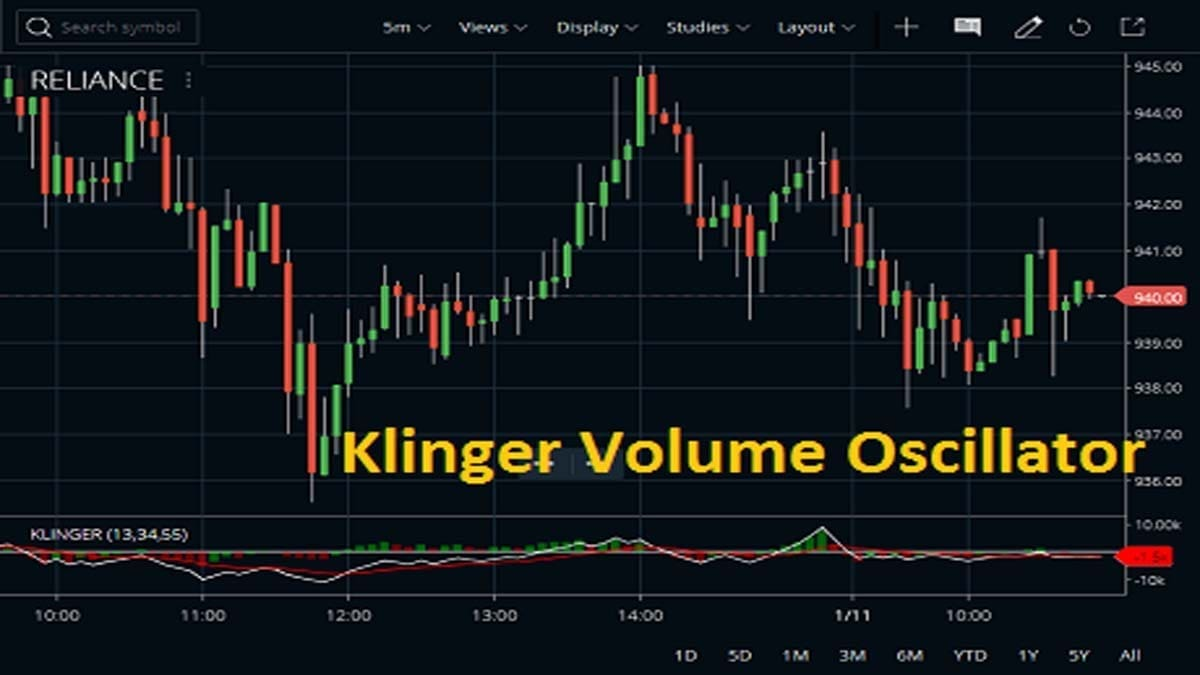Home » Blog » Trading » Technical Indicators » Klinger Volume Oscillator Calculation, Set up

# Klinger Volume Oscillator Calculation, Set upThe Klinger Volume Oscillator (KVO) is a confirmation indicator. Stephen J. Klinger developed the indicator. The Klinger Volume Oscillator uses high, low, close and volume to create a volume force. The popular oscillator helps to forecast an upcoming reversal by using volume and price. Traders can use this to point out short-term fluctuations as well as long-term money flow. So, let’s get into the details of it.

## What is Klinger Volume Oscillator?

The Klinger Volume Oscillator is named after its developer, Stephen J. Klinger. The indicator is able to identify long-term money flow trends. At the same time, it is also sensitive enough to capture short-term reversal in price.

The KVO or KO (Klinger Volume Oscillator) has two main lines, the KVO and Signal lines. Here, the signal line is usually a 13 period EMA of the KVO line. The oscillator works almost similarly like Stochastics.

## How to Calculate Klinger Volume Oscillator?

The calculation of the Klinger Volume Oscillator is slightly critical. To calculate the KVO, the factors require:

• First, note volume for the period along with the high, low, close price.
• Then compare this to the previous period to determine if the trend is positive or negative.
• Calculate (dm= H-L) H=high, L=low
• After that, calculate both the cm value. (cm= cm_1 + dm if Trend = Trend_1) (cm= dm_1+dm if Trend =/= Trend_1)
• The calculation for volume force (VF)
• Then Calculate for VF (Volume Force)
• Lastly, Calculate 34 and 55 period EMAs of VF

So, KVO is complex to calculate, based on the idea of force volume. It accounts for volume, trend (positive/negative), and temp (multiple inputs/statements). The idea reflects how the volume flowing through the stocks is impacting its long-term and short-term price direction.

## How to Use Klinger Volume Oscillator on Charts?

Now, I am going to show you how you can use KVO on different charting platforms. Here, I use Zerodha kite and Upstox pro to set examples. You may use the indicator on other platforms too.

### Use Klinger Volume with Zerodha kite

You can attach KVO in Zerodha Kite from the STUDIES section. You can change the default parameters and colors of the indicator.

A signal line in which a 13-period moving average is used to trigger entry or exit decisions, which is very similar to signals that are created with other indicators such as the ‘moving average convergence divergence’. The short cycle is 34 and the long cycle is 55 of the Klinger Volume Oscillator. You can customize the colors and numbers of the oscillators.

### Use Klinger Volume with Upstox Pro

You can use the oscillator in Upstox Pro almost in the same way as Zerodha Kite. First, select the script on which you wanna work on. Then choose your settings and click on Apply.

## What are the Profit-Making Strategies of the KVO?

There are multiple strategies by which you can make easy profits from the market. So, I am trying to explain some of these important strategies over here.

• Here, the KVO line is originated by subtracting a slow-moving EMA from a fast-moving EMA (Exponential Moving Average).
• When the price is increasing, the volume is added as a positive value there. Reversely, if the price is falling, then the volume is added as a negative value.
• A signal line of KO, which is an EMA of the KO, is plotted to trigger trading signals.
• The KVO has a histogram similar to the MACD indicator. So, this Oscillator can also use divergence to identify when price and volume are not confirming the direction of the move.
• It is a bullish sign when the value of the indicator is heading upward while the price of the security continues to fall.
• In live trading the traders can use other trading tools such as trendlines, moving averages and other technical indicators to confirm the reversal.

### The Uptrend

The uptrend level is when a security price us above 100 periods and MA and Klinger are above zero. Traders could take buy position once the indicator crosses the zero line from below.

Klinger noted that when an asset was in an uptrend, and then suddenly dropped to unusually low levels below zero. After that, it moved above its signal line, this was a favorable long position to take.

### The Downtrend

Now, when the KO crosses the Zero line from the above, it considered downtrend. Traders can short-sell when the market is in the downtrend.

Traders also use the Zero line to mark the transition from an uptrend to a downtrend, or vice versa. If such signals won’t usually agree with price movements, a move above zero helps confirm a rising price. And a drop below zero helps confirm a falling price.

### Divergence

Traders also use KVO divergence to identify when the indicator’s inputs are not confirming the direction of the price move. The bullish indication is when the value of the indicator is heading upward while the price of the security continues to fall. During the Bearish trend, the price is rising but the indicator is falling. The Divergence can also plot with signal line crossovers to generate trades.

## Conclusion

However, it is indeed a very useful oscillator in the technical analysis platform. If we broadly divide the strategies into three sections, we get KVO and signal crossover, Zero crossover, Divergence. It takes volume data at a daily level only. And, this will be effective only on timeframes that are at the daily level or higher (like, daily, weekly, monthly). In order to achieve high accuracy, traders often use this along with other technical tools.

This site uses Akismet to reduce spam. Learn how your comment data is processed.